RS Aggarwal Class 7 Solutions Chapter 8 - Ratio And Proportion

RS Aggarwal Class 7 Chapter 8 - Ratio And Proportion Solutions Free PDF

The mathematical numbers which are used as a method of comparison between two things which are quantifiably similar in terms of units is called as a Ratio. It is represented with the “ : ” symbol and is useful as a metric of measurement for similar things. For example the weight of two bikes can be represented in a ratio form, by considering the different variables.

Weight of Bike A = 100 Kgs

Weight of Bike B = 120 Kgs

Ratio of Bike (A/B)= $\large \frac{100}{120}=\frac{5}{6}=5:6$

Proportions are metrics useful for comparing two or more ratios with each other. In order to check and ensure whether the distributions are equal, we use the concept of Proportions.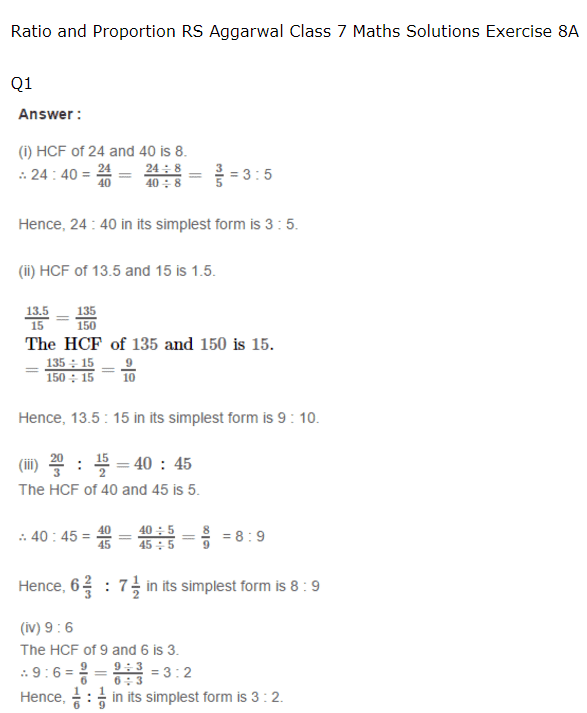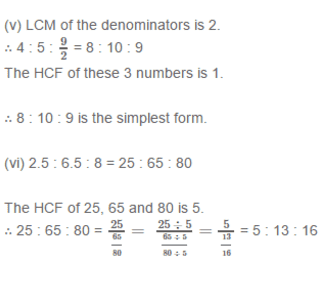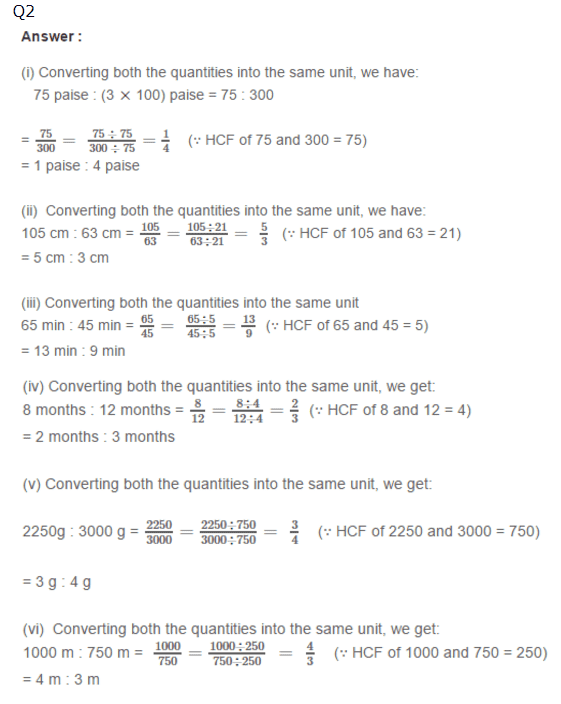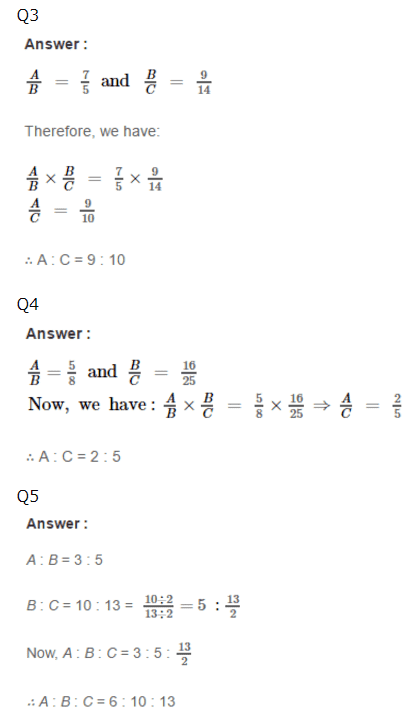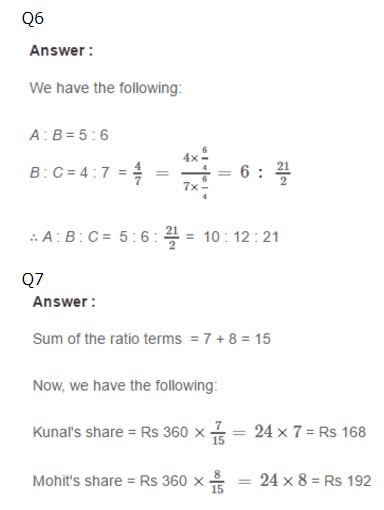Practise This Question

Which of the following uses the most water worldwide?